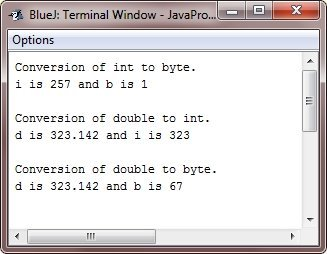# Java Type Casting

Because not all types are compatible, not all type conversions are implicitly permitted. For example, no automatic conversion from "double" to "byte" is defined. Fortunately, a conversion between incompatible types is still possible. You must use a cast to accomplish this, which performs an explicit conversion between the incompatible types.

Although automatic type conversions are useful, they will not meet all of your needs. What if you want to assign an "int" value to a "byte" variable, for example? Because a "byte" is smaller than an "int," this conversion will not be performed automatically. This type of conversion is also known as a narrowing conversion because you are explicitly narrowing the value so that it will fit into the target type.

You must use a cast to create a conversion between the two incompatible types. A cast is nothing more than an explicit type conversion. It takes the following general form:

`(targetType) value`

The "targetType" parameter specifies the type to which the specified value should be converted. For instance, the following code fragment converts an "int" to a "byte." If the integer's value exceeds the range of a byte, it is reduced modulo (the remainder of an integer divided by the range of a byte).

```int a;
byte b;
b = (byte) a;```

When a floating-point value is assigned to an integer type, a different type of conversion occurs: truncation. Integers, as you know, do not have fractional components. As a result, the fractional component is lost when a floating-point value is assigned to an integer type.

For example, if the value 3.14159265358979323846 is assigned to an integer, the resulting value will simply be 3. The 0.14159265358979323846 will have been truncated. Of course, if the size of the whole number component is too large to fit into the target integer type, then that value will be reduced modulo the target type's range.

Java Code
```double d = 3.14159265358979323846;
int i = (int) d;     // typecasting 'double' to 'int'

System.out.println("double value: " + d);
System.out.println("int value: " + i);
```
Output
```double value: 3.141592653589793
int value: 3```

Before closing the discussion on "type casting" in Java, I'm willing to include another example for your understanding.

```public class JavaProgram
{
public static void main(String args[])
{
byte b;
int i = 257;
double d = 323.142;

System.out.println("Conversion of int to byte.");
b = (byte) i;
System.out.println("i is " + i + " and b is " + b);

System.out.println("\nConversion of double to int.");
i = (int) d;
System.out.println("d is " + d + " and i is " + i);

System.out.println("\nConversion of double to byte.");
b = (byte) d;
System.out.println("d is " + d + " and b is " + b);
}
}```

When the above Java program is compiled and run, it will produce the following output:Let me explain the above example step by step, starting with the "conversion of 'int' to 'byte."

#### Conversion of int to byte

```byte b;
int i = 257;
b = (byte) i;```

In this block, we first declare a "byte" variable "b," and an "int" variable "i" with the value of "257." Since the "byte" data type can hold a maximum value of 127 and a minimum value of -128, the value of "i" is out of range for "byte." Therefore, we use explicit typecasting to convert "i" to "byte" and store the result in "b." Since "b" can hold only a smaller range of values than "i," the value of "b" will be truncated and only the last 8 bits of "i" will be stored in "b." In this case, the value of "b" will be -1.

#### Conversion of double to int

```int i;
double d = 323.142;
i = (int) d;```

In this block, we first declare an "int" variable "i," and a "double" variable "d" with the value of "323.142." Since "int" data type can hold only whole numbers and cannot hold decimal places, we use explicit typecasting to convert "d" to "int" and store the result in "i." In this case, the fractional part of "d" will be truncated, and the value of "i" will be "323."

#### Conversion of double to byte

```byte b;
double d = 323.142;
b = (byte) d;```

In this block, we first declare a "byte" variable "b," and a "double" variable "d" with the value of "323.142." Since the "byte" data type can hold a maximum value of 127 and a minimum value of -128, the value of "d" is out of range for "byte." Therefore, we use explicit typecasting to convert "d" to "byte" and store the result in "b." Since "b" can hold only a smaller range of values than "d," the value of "b" will be truncated and only the last 8 bits of "d" will be stored in "b." In this case, the value of "b" will be 63.

Java Online Test

« Previous Tutorial Next Tutorial »